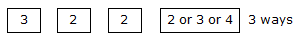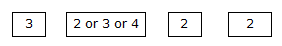# GATE2010-65

9.5k views

Given digits$2, 2, 3, 3, 3, 4, 4, 4, 4$ how many distinct $4$ digit numbers greater than $3000$ can be formed?

1. $50$
2. $51$
3. $52$
4. $54$

edited
0
Can't we use permutation with repetition here?
–1Will this logic be right ?

1
For  1st position either 3 or 4 can be choosen in 2 ways

For 2nd position 2,3,4 can be choosen so 3  ways

For 3rd position 2,3,4 can be choosen so 3 ways

For 4th position 2,3,4 can be choosen so 3 ways

Total=2*3*3*3=54
1
Notice -->

$\binom{9}{4}*4! - \binom{8}{3}*3!$ In this way you will get wrong answer because we are counting same number multiple times.
0
MY first approach was 7*8*7*6=2352 ways .
WHat is wrong in this apporach ? Am i not excluding the repeated parts?
0

We considered selections of ${\color{Red}{\textbf{k items}} }$ out of ${\color{Magenta}{\textbf{n possible options.}} }$

 $\textbf{With repetitions}$ $\textbf{Without repetitions}$ $\textbf{Ordred}$ $\text{Tuples}:n^{k}$ $k-\text{permutations}:\dfrac{n!}{(n-k)!}$ $\textbf{Unordered}$ $\text{Combinations with repetitions}:\binom{k + n - 1}{n-1} = \binom{k + n - 1}{k}$ Also known as Integer Equations - Stars and Bars $\text{Combinations}:\binom{n}{k}$

first place should be occupied by either $3$ or $4.$

Case 1 : First place is occupied by the digit $4$
$4$  _  _  _
now in the set from where we can pick numbers is left with $=\{2,2,3,3,3,4,4,4\}$
if we got $3$ of each digit(which are $2, 3$ and $4$) then number of ways by each of those blanks can be

filled in are $3$ coz we have $3$ choices of digits: $\text{pick } 2, 3$ or $4.$
But we do not have just enough $2's$ to fill all those $3$ spaces with the digit $2.$

$\therefore$ we need to subtract this case where number would be $4222$.
So, total numbers obtained using the numbers in our current set $=1 \times 3 \times 3 \times 3 - 1 = 26$.

The first one is for the digit $4,$ coz its fixed for this case; the subtracted one is for the case $4222$ that can't be made possible.

Case 2: First place is occupied by the digit $3$
$3$  _  _  _
now in the set from where we can pick numbers is left with $=\{2,2,3,3,4,4,4,4\}$
we have enough $4's$ here but lack $3's$ and $2's$ $\therefore$, the cases we need to subtract are $3222$ and $3333$
So, total numbers obtained using the numbers in our current set $=1 \times 3 \times 3 \times 3 - 2 = 25$

both cases are independently capable of giving us the answer, we have =$26 + 25 = 51.$

edited
0
Very  Very Nice Explanation

Let first digit is 3 now rest 3 digits can be from 2,2,3,3,4,4,4,4 but in this no of 2's and 3's are only 2 so 222 and 333 is not possible so the possible no 3*3*3 -2 = 25

In second condition the First no is 4 now rest three digits can be 2,2,3,3,3,4,4,4 so here no of 2's is also two so possible numbers is 3*3*3* -1=26

25+26=51

0
in both the conditions its not clear why 3*3*3-2 and 3*3*3-1 coming.Please explain on this point kindly.

Simplest Approach Ever0
can you explain more?

first digit can be 3 or 4

permutations for remaining 3 places as follows

no digit repeated = 3!

one digit repeated = 3!/2!

non repeating digit can be chosen in 2 ways = (3!/2!)2

repeating digit can be chosen in 3 ways = ((3!/2!)2)3

numbers greater than 3000 but less than 4000 = 3! + ((3!/2!)2)3  = 24 + 1(only 4 can be repeated 3 times

numbers grater than 4000 = 3! + ((3!/2!)2)3  + 2 (since 4 and 3 can be repeated 3 times) = 26

total numbers = 51

edited
0
Explain it
1 vote

Explanation:

The given digits are 2, 2, 3, 3, 3, 4, 4, 4, 4 we have to find the numbers that are greater than 300

The first digit can be 3 or 4 but not 2.

Now, let us fix the first, second and third digits as 3, 2, 2, then the fourth place can be filled in 3 ways.The number of ways is 3 similarly, we fix first third and fourth place as 3, 2 and 2 respectively (4) so the second place can be filled in 3 ways again,The number of ways is 3

Now, we fix first, second and fourth, previous cases and we obtain the same result.

The number of ways is 3 so, the total number of ways is 9 similarly this can done by fixing the numbers as 3 and 4 (instead of 2) and thereby we obtain the a ways each

The number of numbers starting with 3 is 27

Similarly by taking 4 as the first digit we get 27 numbers

The number of numbers that are greater than 3000 is 27 + 27 = 54

But, 3222, 4222, is not possible as there are only two 2's, 3333 is not possible as there are only three 3's

The total number of numbers that are greater than 3000 is 54 - 3 = 51

Hope it helps u.:)

1 vote

Numbers are 2,2,3,3,3,4,4,4,4

As it is 4 digit number first place must be 3,or 4

If 1st place is 3

2nd place can be fill up from any of rest 8 numbers.

3rd place can be fill up with any of rest 7 numbers

4 th place can be fill up with any of rest 6 numbers

So, total numbers $8\times 7\times 6=336$

If 1st place is 4

Similarly it also can be fill with 336 numbers

So, total $2\times 336$=672

2
what if second and third places are filled with same digit? You are double counting them. Similarly for all positions.
0
Plz can any one explain why this method is going to be wrong
0
if you used all the 3's and all the 4's in the first place you will end up forming the same number over and over again, and the question was to count the distinct numbers that can be formed. Instead you should use one 3 and one 4 to get distinct numbers.

For instance, suppose I used the first 3 for the first place and the first 4 for the second place, then the 2 then the 2, I'll end up with the number 3422 right? And if I used the second 3 and the second 4 then 2 then 2, I'll also end up with the same number 3422. Which we don't want to count it another time. (The keyword is Distinct).

## Related questions

1
2.9k views
$5$ skilled workers can build a wall in $20$ days; $8$ semi-skilled workers can build a wall in $25$ days; $10$ unskilled workers can build a wall in $30$ days. If a team has $2$ skilled, $6$ semi-skilled and $5$ unskilled workers, how long it will take to build the wall? $20$ days $18$ days $16$ days $15$ days
Hari(H), Gita(G), Irfan(I) and Saira(S) are siblings (i.e., brothers and sisters). All were born on 1st January. The age difference between any two successive siblings (that is born one after another) is less than three years. Given the following facts: Hari's age + Gita ... and Saira is not the youngest. There are no twins. In what order they were born (oldest first)? $HSIG$ $SGHI$ $IGSH$ $IHSG$
If $137 + 276 = 435$ how much is $731+672?$ $534$ $1403$ $1623$ $1513$
$25$ persons are in a room. $15$ of them play hockey, $17$ of them play football and $10$ of them play both hockey and football. Then the number of persons playing neither hockey nor football is: $2$ $17$ $13$ $3$